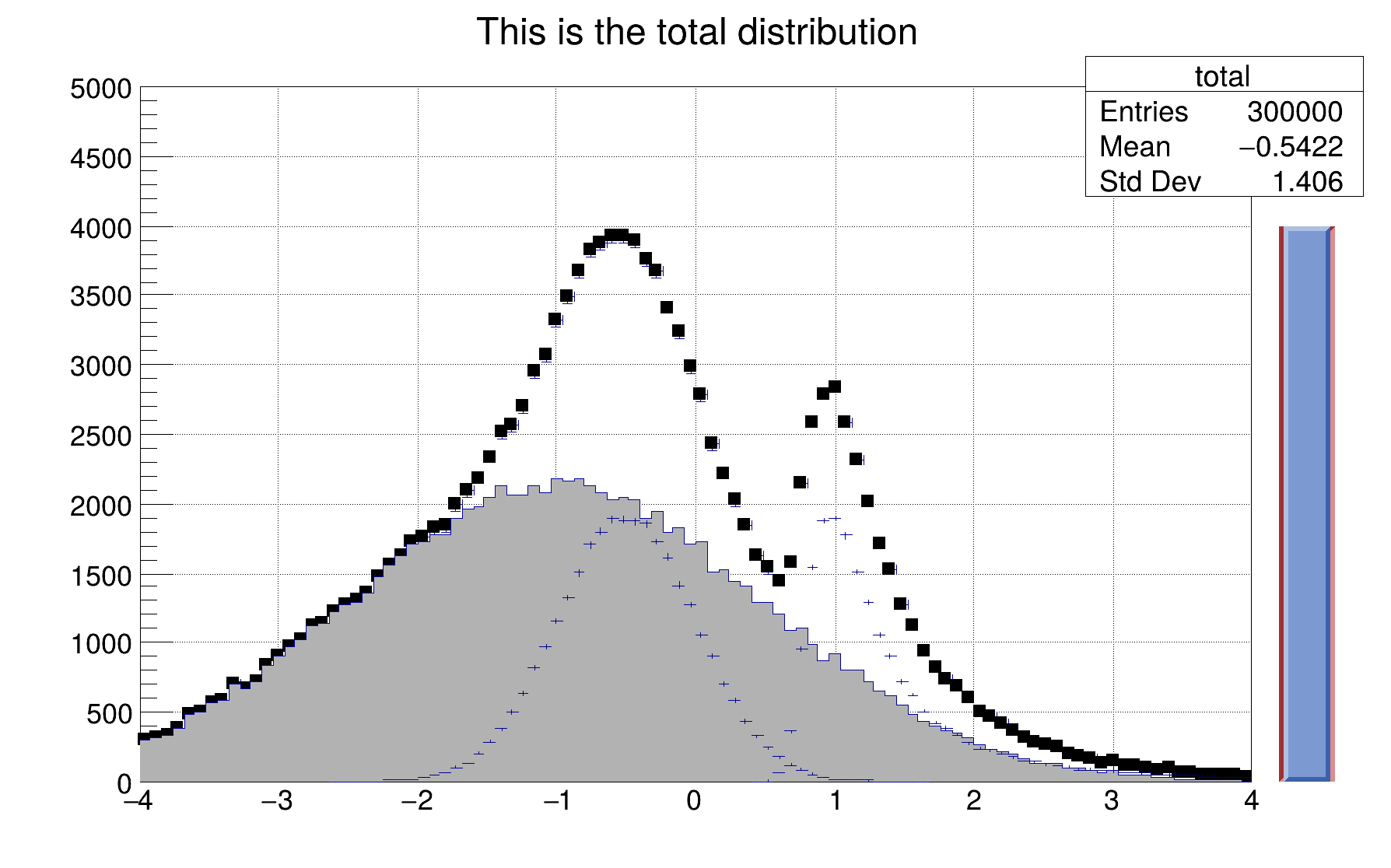ROOT   Reference Guide
Searching...
No Matches
hsumTimer.C File Reference

## Detailed Description

Simple example illustrating how to use the C++ interpreter to fill histograms in a loop and show the graphics results This program is a variant of the tutorial "hsum". It illustrates the use of Timers.Float_t progressRatio = 0.;
TSlider *slider = nullptr;
TCanvas *c1 = nullptr;
void hsumUpdate()
{
// called when Timer times out
if (slider) slider->SetRange(0., progressRatio);
c1->Modified();
c1->Update();
}
void hsumTimer(Int_t nfill=100000)
{
c1 = new TCanvas("c1","The HSUM example",200,10,600,400);
c1->SetGrid();
// Create some histograms.
auto total = new TH1F("total","This is the total distribution",100,-4,4);
auto main = new TH1F("main","Main contributor",100,-4,4);
auto s1 = new TH1F("s1","This is the first signal",100,-4,4);
auto s2 = new TH1F("s2","This is the second signal",100,-4,4);
total->Sumw2(); // store the sum of squares of weights
total->SetMarkerStyle(21);
total->SetMarkerSize(0.7);
main->SetFillColor(16);
s1->SetFillColor(42);
s2->SetFillColor(46);
total->SetMaximum(nfill/20.);
total->Draw("e1p");
main->Draw("same");
s1->Draw("same");
s2->Draw("same");
c1->Update();
slider = new TSlider("slider","test",4.2,0,4.6,0.8*total->GetMaximum(),38);
slider->SetFillColor(46);
// Create a TTimer (hsumUpdate called every 300 msec)
TTimer timer("hsumUpdate()",300);
timer.TurnOn();
// Fill histograms randomly
Float_t xs1, xs2, xmain;
for (Int_t i=0; i<nfill; i++) {
progressRatio = i * 1. / nfill;
if (gSystem->ProcessEvents()) break;
xmain = gRandom->Gaus(-1,1.5);
xs1 = gRandom->Gaus(-0.5,0.5);
xs2 = gRandom->Landau(1,0.15);
main->Fill(xmain);
s1->Fill(xs1,0.3);
s2->Fill(xs2,0.2);
total->Fill(xmain);
total->Fill(xs1,0.3);
total->Fill(xs2,0.2);
}
timer.TurnOff();
hsumUpdate();
}
int main()
Definition Prototype.cxx:12
#define s1(x)
Definition RSha256.hxx:91
int Int_t
Definition RtypesCore.h:45
float Float_t
Definition RtypesCore.h:57
static unsigned int total
R__EXTERN TRandom * gRandom
Definition TRandom.h:62
R__EXTERN TSystem * gSystem
Definition TSystem.h:560
virtual void SetFillColor(Color_t fcolor)
Set the fill area color.
Definition TAttFill.h:37
The Canvas class.
Definition TCanvas.h:23
1-D histogram with a float per channel (see TH1 documentation)}
Definition TH1.h:578
virtual Double_t Gaus(Double_t mean=0, Double_t sigma=1)
Samples a random number from the standard Normal (Gaussian) Distribution with the given mean and sigm...
Definition TRandom.cxx:274
virtual void SetSeed(ULong_t seed=0)
Set the random generator seed.
Definition TRandom.cxx:608
virtual Double_t Landau(Double_t mean=0, Double_t sigma=1)
Generate a random number following a Landau distribution with location parameter mu and scale paramet...
Definition TRandom.cxx:380
A specialized TPad including a TSliderBox object.
Definition TSlider.h:17
virtual void SetRange(Double_t xmin=0, Double_t xmax=1)
Set Slider range in [0,1].
Definition TSlider.cxx:183
virtual Bool_t ProcessEvents()
Process pending events (GUI, timers, sockets).
Definition TSystem.cxx:403
Handles synchronous and a-synchronous timer events.
Definition TTimer.h:51
return c1
Definition legend1.C:41

Definition in file hsumTimer.C.